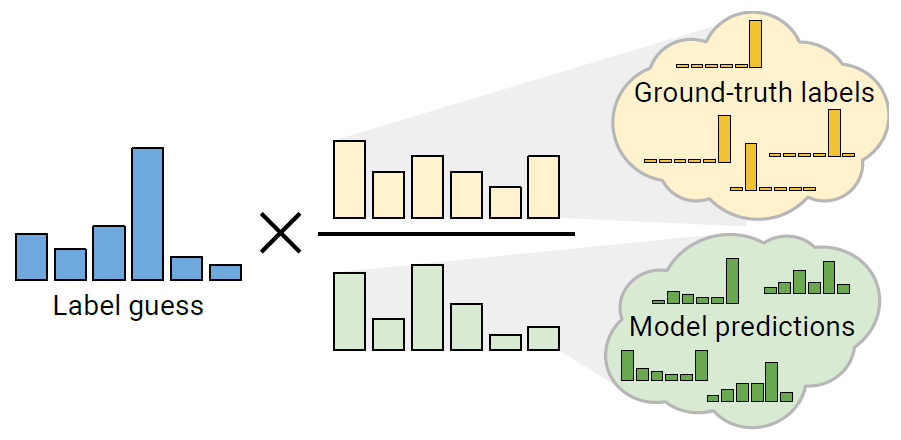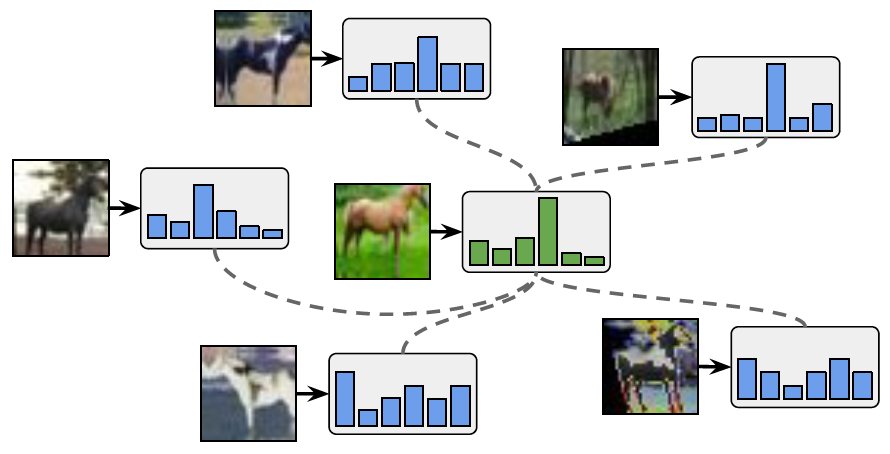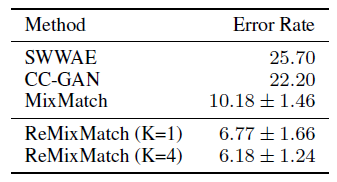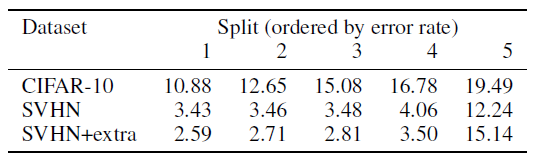# Review — ReMixMatch: Semi-Supervised Learning with Distribution Alignment and Augmentation Anchoring

## ReMixMatch, Improving MixMatch by Introducing Two New Techniques

ReMixMatch: Semi-Supervised Learning with Distribution Alignment and Augmentation Anchoring
ReMixMatch, by Google Research ,and Google Cloud AI
2020 ICLR, Over 400 Citations (Sik-Ho Tsang @ Medium)
Semi-Supervised Learning, Image Classification

• ReMixMatch improves MixMatch by introducing two new techniques:
1. Distribution alignment encourages the marginal distribution of predictions on unlabeled data to be close to the marginal distribution of ground-truth labels.
2. Augmentation anchoring feeds multiple strongly augmented versions of an input into the model and encourages each output to be close to the prediction for a weakly-augmented version of the same input.

# Outline

1. MixMatch Brief Review
2. Distribution Alignment
3. Augmentation Anchoring
4. Putting It All Together
5. Experimental Results

# 1. MixMatch Brief Review

• MixMatch generates “guessed labels” for each unlabeled example, and then using fully-supervised techniques to train on the original labeled data along with the guessed labels for the unlabeled data.
• MixMatch first produces K weakly augmented versions of each unlabeled datapoint. A guessed label is generated by averaging the K predictions.
• Sharpening is used reduce the entropy of the label distribution.
• Pairs of examples (x1, p1), (x2, p2) from the combined set of labeled examples and unlabeled examples with label guesses are fed into the mixup.
• A standard cross-entropy loss is used for labeled data, whereas the loss for unlabeled data is computed using a mean square error.
• (Please feel free to read MixMatch if interested.)

# 2. Distribution AlignmentDistribution Alignment: Guessed label distributions are adjusted according to the ratio of the empirical ground-truth class distribution divided by the average model predictions on unlabeled data.

Distribution Alignment enforces that the aggregate of predictions on unlabeled data matches the distribution of the provided labeled data.

• A running average of the model’s predictions on unlabeled data is maintained, which is referred to as ~p(y).
• Given the model’s prediction q=pmodel(y|u,θ) on an unlabeled example u, q is scaled by the ratio p(y)/~p(y) and then renormalized to form a valid probability distribution:
• where:
• Then, ~q is used as the label guess for u.
• In practice, ~p(y) is computed as the moving average of the model’s predictions on unlabeled examples over the last 128 batches. And the marginal class distribution p(y) is estimated based on the labeled examples seen during training.

# 3. Augmentation AnchoringAugmentation Anchoring: The prediction for a weakly augmented image (green, middle) is used as the target for predictions on strong augmentations of the same image (blue).
• A variant of AutoAugment or RandAugment, CTAugment, is proposed.
• It is hypothesized that MixMatch with AutoAugment is unstable: MixMatch averages the prediction across K augmentations. Stronger augmentation can result in disparate predictions, so their average may not be a meaningful target.

Instead, given an unlabeled input, an “anchor” is first generated by applying weak augmentation to it. Then, K strongly-augmented versions of the same unlabeled input are generated using CTAugment (described below).

The guessed label (after applying distribution alignment and sharpening) is used as the target for all of the K strongly-augmented versions of the image.

## 3.1. Control Theory Augment (CTAugment)

CTAugment only samples augmentations that fall within the network tolerance.

• Let m be the vector of bin weights for some distortion parameter for some transformation. At the beginning of training, all magnitude bins are initialized to have a weight set to 1.
• At each training step, for each image two transformations are sampled uniformly at random.
• A modified set of bin weights ^m is produced where ^mi = mi if mi>0.8 and ^mi=0 otherwise, and magnitude bins are sampled from Categorical(Normalize(^m)).
• The resulting transformations are applied to a labeled example x with label p to obtain an augmented version ^x.
• Then, the extent to which the model’s prediction matches the label is measured as:
• The weight for each sampled magnitude bin is updated as:
• where ρ=0.99 is a fixed exponential decay hyperparameter.

To be brief, CTAugment is a kind of RandAugment which samples the magnitude of transformation.

# 4. Putting It All Together

• The main purpose of this algorithm is to produce the collections X’ and U’, consisting of augmented labeled and unlabeled examples with mixup applied. The labels and label guesses in X’ and U are fed into standard cross-entropy loss terms against the model’s predictions.
• As shown above, ^U1 is also outputted, which consists of a single heavily-augmented version of each unlabeled image and its label guesses without mixup applied. ^U1 is used in two additional loss terms which provide a mild boost in performance in addition to improved stability: Pre-mixup unlabeled loss and Rotation loss.
1. Pre-mixup unlabeled loss: the guessed labels and predictions for example in ^U1 are fed as-is into a separate cross-entropy loss term.
2. Rotation loss: Idea from self-supervised learning RotNet is used where each ^U1 image is rotated and the model needs to predict the rotation amount.
• In total, the ReMixMatch loss is:
• In practice, λr=λ^U1=0.5. λU=1.5. T=0.5 for sharpening, and Beta=0.75 for mixup. K=8. The final model as an exponential moving average over the trained model weights with a decay of 0.999.

# 5. Experimental Results

Realistic Semi-Supervised Learning (Oliver NeurIPS’18) recommendations for performing semi-supervised learning (SSL) evaluations.

## 5.1. CIFAR-10 & SHVN

ReMixMatch sets the new state-of-the-art for all numbers of labeled examples.

• Most importantly, ReMixMatch is 16× more data efficient than MixMatch (e.g., at 250 labeled examples ReMixMatch has identical accuracy compared to MixMatch at 4,000).
• ReMixMatch reaches state-of-the-art at 250 labeled examples, and within the margin of error for state-of-the-art otherwise.

## 5.2. STL-10STL-10 error rate using 1000-label splits

Using the same WRN-37–2 network (23.8 million parameters), the error rate is reduced by a factor of two compared to MixMatch.

## 5.3. Towards Few-Shot LearningSorted error rate of ReMixMatch with 40 labeled examples
• High variance is to be expected when choosing so few labeled examples at random.
• On CIFAR-10, ReMixMatch obtains a median-of-five error rate of 15.08%.
• On SVHN ReMixMatch reaches 3.48% error.
• On SVHN with the “extra” dataset, ReMixMatch reaches 2.81% error.

ReMixMatch is able to work in extremely low-label settings.

## 5.4. Ablation Study

• Removing the rotation loss reduces accuracy at 250 labels by only 0.14 percentage points, but it is found that in the 40-label setting, rotation loss is necessary to prevent collapse.
• Changing the cross-entropy loss on unlabeled data to an L2 loss as used in MixMatch hurts performance dramatically, as does removing either of the augmentation components.

This validates using augmentation anchoring in place of the consistency regularization mechanism of MixMatch.

(Later on, there is another approach called FixMatch proposed, which outperforms ReMixMatch. I hope I can review it in the coming future.)

## Reference

[2020 ICLR] [ReMixMatch]
ReMixMatch: Semi-Supervised Learning with Distribution Alignment and Augmentation Anchoring

## Pretraining or Weakly/Semi-Supervised Learning

2004 … 2019 [VAT] [Billion-Scale] [Label Propagation] [Rethinking ImageNet Pre-training] [MixMatch] [SWA & Fast SWA] [S⁴L] 2020 [BiT] [Noisy Student] [SimCLRv2] [UDA] [ReMixMatch]

--

--